# sklearn.semi_supervised.LabelSpreading¶

class sklearn.semi_supervised.LabelSpreading(kernel='rbf', *, gamma=20, n_neighbors=7, alpha=0.2, max_iter=30, tol=0.001, n_jobs=None)[source]

This model is similar to the basic Label Propagation algorithm, but uses affinity matrix based on the normalized graph Laplacian and soft clamping across the labels.

Read more in the User Guide.

Parameters:
kernel{‘knn’, ‘rbf’} or callable, default=’rbf’

String identifier for kernel function to use or the kernel function itself. Only ‘rbf’ and ‘knn’ strings are valid inputs. The function passed should take two inputs, each of shape (n_samples, n_features), and return a (n_samples, n_samples) shaped weight matrix.

gammafloat, default=20

Parameter for rbf kernel.

n_neighborsint, default=7

Parameter for knn kernel which is a strictly positive integer.

alphafloat, default=0.2

Clamping factor. A value in (0, 1) that specifies the relative amount that an instance should adopt the information from its neighbors as opposed to its initial label. alpha=0 means keeping the initial label information; alpha=1 means replacing all initial information.

max_iterint, default=30

Maximum number of iterations allowed.

tolfloat, default=1e-3

Convergence tolerance: threshold to consider the system at steady state.

n_jobsint, default=None

The number of parallel jobs to run. None means 1 unless in a joblib.parallel_backend context. -1 means using all processors. See Glossary for more details.

Attributes:
X_ndarray of shape (n_samples, n_features)

Input array.

classes_ndarray of shape (n_classes,)

The distinct labels used in classifying instances.

label_distributions_ndarray of shape (n_samples, n_classes)

Categorical distribution for each item.

transduction_ndarray of shape (n_samples,)

Label assigned to each item during fit.

n_features_in_int

Number of features seen during fit.

New in version 0.24.

feature_names_in_ndarray of shape (n_features_in_,)

Names of features seen during fit. Defined only when X has feature names that are all strings.

New in version 1.0.

n_iter_int

Number of iterations run.

LabelPropagation

Unregularized graph based semi-supervised learning.

References

Dengyong Zhou, Olivier Bousquet, Thomas Navin Lal, Jason Weston, Bernhard Schoelkopf. Learning with local and global consistency (2004)

Examples

>>> import numpy as np
>>> from sklearn import datasets
>>> rng = np.random.RandomState(42)
>>> random_unlabeled_points = rng.rand(len(iris.target)) < 0.3
>>> labels = np.copy(iris.target)
>>> labels[random_unlabeled_points] = -1
>>> label_prop_model.fit(iris.data, labels)


Methods

 fit(X, y) Fit a semi-supervised label propagation model to X. get_params([deep]) Get parameters for this estimator. Perform inductive inference across the model. Predict probability for each possible outcome. score(X, y[, sample_weight]) Return the mean accuracy on the given test data and labels. set_params(**params) Set the parameters of this estimator.
fit(X, y)[source]

Fit a semi-supervised label propagation model to X.

The input samples (labeled and unlabeled) are provided by matrix X, and target labels are provided by matrix y. We conventionally apply the label -1 to unlabeled samples in matrix y in a semi-supervised classification.

Parameters:
Xarray-like of shape (n_samples, n_features)

Training data, where n_samples is the number of samples and n_features is the number of features.

yarray-like of shape (n_samples,)

Target class values with unlabeled points marked as -1. All unlabeled samples will be transductively assigned labels internally, which are stored in transduction_.

Returns:
selfobject

Returns the instance itself.

get_params(deep=True)[source]

Get parameters for this estimator.

Parameters:
deepbool, default=True

If True, will return the parameters for this estimator and contained subobjects that are estimators.

Returns:
paramsdict

Parameter names mapped to their values.

predict(X)[source]

Perform inductive inference across the model.

Parameters:
Xarray-like of shape (n_samples, n_features)

The data matrix.

Returns:
yndarray of shape (n_samples,)

Predictions for input data.

predict_proba(X)[source]

Predict probability for each possible outcome.

Compute the probability estimates for each single sample in X and each possible outcome seen during training (categorical distribution).

Parameters:
Xarray-like of shape (n_samples, n_features)

The data matrix.

Returns:
probabilitiesndarray of shape (n_samples, n_classes)

Normalized probability distributions across class labels.

score(X, y, sample_weight=None)[source]

Return the mean accuracy on the given test data and labels.

In multi-label classification, this is the subset accuracy which is a harsh metric since you require for each sample that each label set be correctly predicted.

Parameters:
Xarray-like of shape (n_samples, n_features)

Test samples.

yarray-like of shape (n_samples,) or (n_samples, n_outputs)

True labels for X.

sample_weightarray-like of shape (n_samples,), default=None

Sample weights.

Returns:
scorefloat

Mean accuracy of self.predict(X) w.r.t. y.

set_params(**params)[source]

Set the parameters of this estimator.

The method works on simple estimators as well as on nested objects (such as Pipeline). The latter have parameters of the form <component>__<parameter> so that it’s possible to update each component of a nested object.

Parameters:
**paramsdict

Estimator parameters.

Returns:
selfestimator instance

Estimator instance.

## Examples using sklearn.semi_supervised.LabelSpreading¶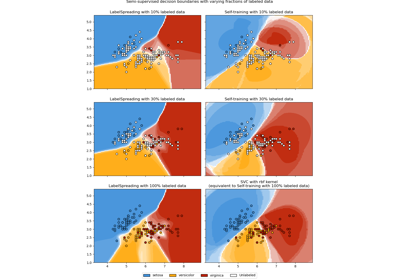Decision boundary of semi-supervised classifiers versus SVM on the Iris dataset

Decision boundary of semi-supervised classifiers versus SVM on the Iris dataset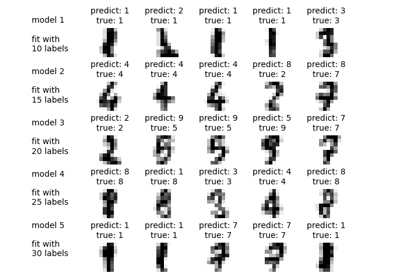Label Propagation digits active learning

Label Propagation digits active learning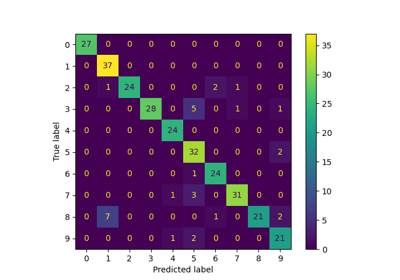Label Propagation digits: Demonstrating performance

Label Propagation digits: Demonstrating performance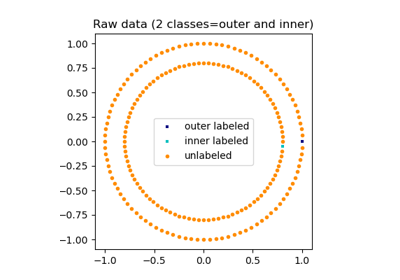Label Propagation learning a complex structure

Label Propagation learning a complex structure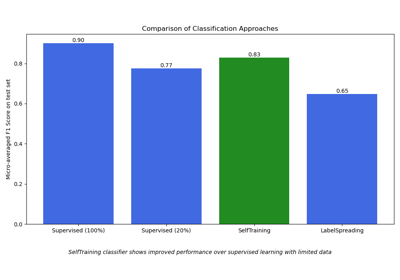Semi-supervised Classification on a Text Dataset

Semi-supervised Classification on a Text Dataset